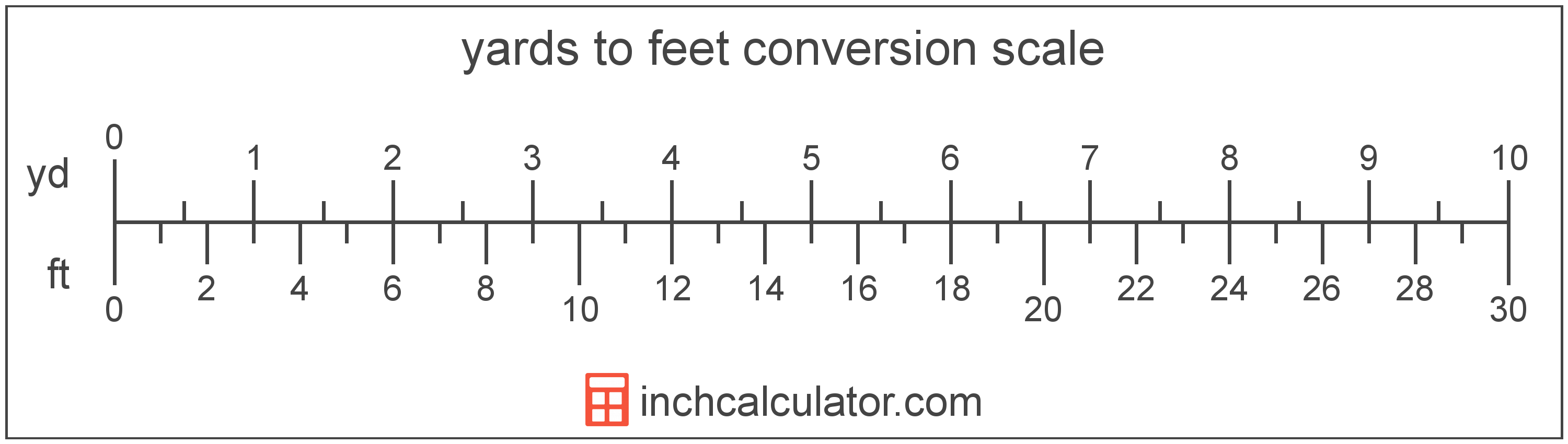# Feet to Yards Conversion

Enter the length in feet below to get the value converted to yards.

ft
in
Results in Yards:3 ft = 1 yd
Do you want to convert yards to feet?

## How to Convert Feet to YardsTo convert a foot measurement to a yard measurement, divide the length by the conversion ratio.

Since one yard is equal to 3 feet, you can use this simple formula to convert:

yards = feet ÷ 3

The length in yards is equal to the feet divided by 3.

For example, here's how to convert 5 feet to yards using the formula above.
5' = (5 ÷ 3) = 1.666667 yd

Our inch fraction calculator can add feet and yards together, and it also automatically converts the results to US customary, imperial, and SI metric values.

## Feet

The foot is a unit of linear length measure equal to 12 inches or 1/3 of a yard. Because the international yard is legally defined to be equal to exactly 0.9144 meters, one foot is equal to 0.3048 meters.

The foot is a US customary and imperial unit of length. Feet can be abbreviated as ft; for example, 1 foot can be written as 1 ft.

Feet can also be denoted using the symbol, otherwise known as a prime, though a single-quote (') is often used instead of the prime symbol for convenience. Using the prime symbol, 1 ft can be written as 1′.

The foot is most commonly measured using either a standard 12" ruler or a tape measure, though there are many other measuring devices available. Feet are sometimes referred to as linear feet, which are simply the measurement of length in feet.

You might be interested in our feet and inches calculator, which can add feet with inches, centimeters, or meters.

## Yards

The yard is a unit of length measurement equal to 3 feet or 36 inches. The international yard is legally defined to be equal to exactly 0.9144 meters.

The yard is a US customary and imperial unit of length. Yards can be abbreviated as yd; for example, 1 yard can be written as 1 yd.

We recommend using a ruler or tape measure for measuring length, which can be found at a local retailer or home center. Rulers are available in imperial, metric, or combination with both values, so make sure you get the correct type for your needs.

Need a ruler? Try our free downloadable and printable rulers, which include both imperial and metric measurements.

## Foot to Yard Conversion Table

Foot measurements converted to yards
Feet Yards
1' 0.333333 yd
2' 0.666667 yd
3' 1 yd
4' 1.3333 yd
5' 1.6667 yd
6' 2 yd
7' 2.3333 yd
8' 2.6667 yd
9' 3 yd
10' 3.3333 yd
11' 3.6667 yd
12' 4 yd
13' 4.3333 yd
14' 4.6667 yd
15' 5 yd
16' 5.3333 yd
17' 5.6667 yd
18' 6 yd
19' 6.3333 yd
20' 6.6667 yd
21' 7 yd
22' 7.3333 yd
23' 7.6667 yd
24' 8 yd
25' 8.3333 yd
26' 8.6667 yd
27' 9 yd
28' 9.3333 yd
29' 9.6667 yd
30' 10 yd
31' 10.33 yd
32' 10.67 yd
33' 11 yd
34' 11.33 yd
35' 11.67 yd
36' 12 yd
37' 12.33 yd
38' 12.67 yd
39' 13 yd
40' 13.33 yd

## References

1. National Institute of Standards and Technology, U.S. Survey Foot: Revised Unit Conversion Factors, https://www.nist.gov/pml/us-surveyfoot/revised-unit-conversion-factors
2. National Bureau of Standards, Refinement of Values for the Yard and the Pound, https://www.ngs.noaa.gov/PUBS_LIB/FedRegister/FRdoc59-5442.pdf